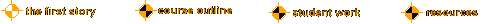Note: the following has been abstracted from the Grolier Encyclopedia.

### Non-Euclidean Geometry

Non-Euclidean Geometry refers to two geometries--hyperbolic geometry and elliptic geometry. These geometries are based on the first four postulates of Euclid but assume alternatives to his parallel postulate instead of assuming the postulate itself. The parallel postulate is equivalent to an assumption that there is exactly one line that is parallel to a given line m and contains a given point that is not a point of the line m. Hyperbolic geometry may be obtained by replacing Euclid's parallel postulate by an assumption that there are at least two lines that are parallel to a given line m and contain a given point that is not a point of the line m. Elliptic geometry may be obtained by replacing Euclid's parallel postulate by an assumption that no line exists that is parallel to a given line m and contains a point that is not a point of the line m. The three cases--(1) exactly one line (Euclidean geometry), (2) at least two lines (hyberbolic geometry), and (3) no lines (elliptic geometry)--represent all possibilities. Hence exactly two non-Euclidean geometries exist even though many geometries exist that are not Euclidean.

#### Comparisons of the Geometries

The sum of the measures of the angles of a triangle is 180 deg in Euclidean Geometry, less than 180 deg in hyperbolic geometry, and more than 180 deg in elliptic geometry. The area of a triangle in hyperbolic geometry is proportional to the deficiency of its angle sum from 180 deg, while the area of a triangle in elliptic geometry is proportional to the excess of its angle sum over 180 deg. In Euclidean geometry all triangles have an angle sum of 180 deg irrespective of area. Thus, similar triangles with different areas can exist in Euclidean geometry. This kind of occurrence is not possible in hyperbolic or elliptic geometry.

In two-dimensional geometries, lines that are perpendicular to the same given line are parallel in Euclidean geometry, are neither parallel nor intersecting in hyperbolic geometry, and intersect at the pole of the given line in elliptic geometry. The appearance of the lines as straight or curved depends on the postulates for the space.

#### Historical Development

Euclid postponed the use of his parallel postulate as long as possible and probably was a bit uneasy about it. For the next 2,000 years numerous attempts were made to prove the statement as a theorem. Many of the attempts involved statements that are now recognized as equivalent to the parallel postulate. These statements include the following: (1) Parallel lines exist, and any two lines cut by a transversal are no more parallel on one side of the transversal than on the other side (2d century AD). (2) Parallel lines exist, and if a line intersects one of two parallel lines, it will intersect the other also (5th century). (3) The sum of the measures of the angles of any triangle is equal to a straight angle (13th century). (4) Given any triangle, a similar triangle of any size whatever may be constructed (17th century).

A proof of the parallel postulate by reductio ad absurdum was attempted by Girolamo Saccheri (1667-1733) in his work Euclid Freed of Every Flaw. Saccheri constructed a quadrilateral ABCD with congruent sides AD and BC and with right angles at A and B. He proved that the angles at C and D were congruent and considered three hypotheses depending on whether the angles C and D were acute angles (hyperbolic geometry), obtuse angles (elliptic geometry), or right angles (Euclidean geometry). Unfortunately, Saccheri's reasons for rejecting the acute-angle hypothesis and the obtuse-angle hypothesis are not acceptable by present standards. However, Saccheri's work provided foundations for successful independent efforts by Janos Bolyai and Nicolai Lobachevsky in the development of hyperbolic geometry and of Georg Riemann in the development of elliptic geometry. Carl F. Gauss appears to have recognized the existence of non-Euclidean geometries. Arthur Cayley and Felix Klein developed distance functions (metrics) for these geometries. In the 19th and 20th centuries the non-Euclidean geometries have been accepted as consistent geometries and have been extended to three and more and to fractional dimensions.

#### The Geometry of the Universe

The recognition of the existence of the non-Euclidean geometries as mathematical systems was resisted by many people who--with an almost religious fervor--proclaimed that Euclidean geometry was the one and only geometry. Such attitudes reflect a failure to recognize that a geometry is a mathematical system that is determined by its assumptions. The truth of the geometry in the sense of representing our universe is a matter of observation. At the present time mathematicians are not even sure which of the three geometries provides the best representation of the entire universe. They do know that Euclidean geometry provides an excellent representation for the part of the universe that we inhabit. Unfortunately, all observed measurements are approximate. Thus, the sum of the angles of a triangle might someday be proved different from 180 deg, but this possibility can never be proved by measurement.

The future of the universe is expected to be determined by whatever is the actual geometry of the universe. According to current theories in Cosmology, if the geometry is hyperbolic, the universe will expand indefinitely; if the geometry is Euclidean, the universe will expand indefinitely at escape velocity; and if the geometry is elliptic, the expansion of the universe will coast to a halt, and then the universe will start to shrink, possibly to explode again.